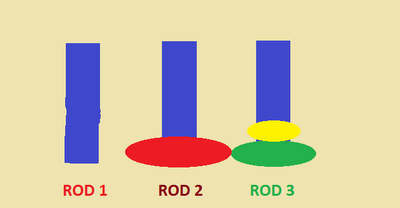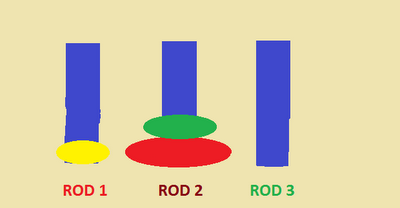## Monday, 3 December 2012

### Tower of Hanoi Problem

The Tower of Hanoi Problem is a mathematical problem. There are three rods and on one of the rods there are some disks of unique size. The problem is to move the disks from one rod to the second one, using the third rod as an intermediary. But while moving the disks the following rules should not be violated.
• Only one disk can be moved at a time.
• A larger disk should not be placed on top of a smaller disk.
• Only the disk on the top of a rod can be moved.
The number of moves required to solve the Tower of Hanoi Problem with N Disks is 2N-1.
Unlike many other problems discussed here, the time complexity of the Tower of Hanoi problem is not polynomial. It takes an exponential time O(2n) to solve the problem. The figures given below illustrates the solution for the Tower of Hanoi problem with three disks. It takes 7 moves to solve the problem.Initial PositionAfter Move 1After Move 2After Move 3After Move 4After Move 5After Move 6After Move 7